# HSSlive: Plus One & Plus Two Notes & Solutions for Kerala State Board

## BSEB Class 12 Physics Dual Nature of Radiation and Matter Textbook Solutions PDF: Download Bihar Board STD 12th Physics Dual Nature of Radiation and Matter Book AnswersBSEB Class 12 Physics Dual Nature of Radiation and Matter Textbook Solutions PDF: Download Bihar Board STD 12th Physics Dual Nature of Radiation and Matter Book Answers

## Bihar Board Class 12th Physics Dual Nature of Radiation and Matter Books Solutions

 Board BSEB Materials Textbook Solutions/Guide Format DOC/PDF Class 12th Subject Physics Dual Nature of Radiation and Matter Chapters All Provider Hsslive

2. Click on the Bihar Board Class 12th Physics Dual Nature of Radiation and Matter Answers.
3. Look for your Bihar Board STD 12th Physics Dual Nature of Radiation and Matter Textbooks PDF.

Find below the list of all BSEB Class 12th Physics Dual Nature of Radiation and Matter Textbook Solutions for PDF’s for you to download and prepare for the upcoming exams:

Question 1. Find the (a) maximum frequency, and (b) minimum wavelength of X-rays produced by 30 kVelectrons. Answer: Here, V = potential difference = 30 kV = 30,000 V. (a) Let v max = maximum frequency of X-rays produced = ? Using the formula E = hv = eV, we get v max = 𝑒𝑉ℎ Here, h = 6.62 x 10-34Js, e = 1.6 x 10-19 C. v max = 1.6×10−19×3×1046.62×10−34 = 7.24 x 10-18 Hz.

(b) Let λmin = minimum wavelength of X-rays produced = ? Using the relation, c = vλ., we get λmin = 𝑐𝑣max where c = 3 x 108 ms-1, vmax = 7.24 x 10-18 Hz λmin = 3×1087.24×1018 = 4.14 x 1011 m = 4.14×10−1010 = 0.414 x 10-10m = 0.414 Å = 0.0414 nm (∴1 Å = 10-10 m)

Question 2. The work function of caesium metal is 2.14 eV. When light of frequency 6 x 1014 Hz is incident on the metal surface, photoemission of electrons occurs. What is the (a) maximum kinetic energy of the emitted electrons. (b) Stopping potential, and (c) maximum speed of the emitted photoelectrons? Answer: Here, ω = work function of Caesium metal = 2.14 eV = 2.14 x 1.6 x 10-19J. v = frequency of incident light = 6x 10-14Hz. h = Planck’s constant = 6.62 x 10-34 Js. ∴ Energy of incident light = hv = 6.62 x 10-34 x 6 x 1014 J.

(a) Emax = maximum kinetic energy of photo electrons emitted = ? Using Einstein’s photoelectric equation, hv = ω + Emax, we get Emax = hv – w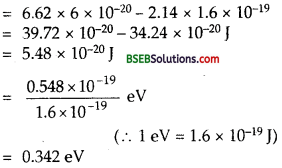(b) V0 = Stopping potential =? By definition, eV0 = Emax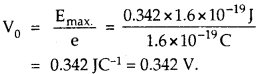(c) Let vmax = maximum speed of emitted photo electron = ? M = mass of electron = 9.1 x 10-31 kg Using formula,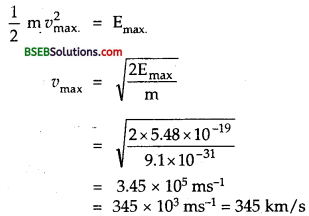Question 3. The phtoelectric cut-off voltage in a certain experiment is 1.5 V. What is the maximum kinetic energy of photoelectrons emitted? Answer: Here, VQ = cut off voltage or stopping potential = 1.5 V Emax= Maximum Kinetic energy emitted = ? e = charge of an electron = 1.6 x 10-19 C Using the relation, KE = Emax = eV0we get Emax = 1.6 X 10-19 x 1.5 = 2.4 x 10-19J

Question 4. Monochromatic light of wavelength 632.8 nm is produced by a helium-neon laser. The power emitted is 9.42 mW. (a) Find the energy and momentum of each photon in the light beam. (b) How many photons per second, on the average, arrive at a target irradiated by this beam? (Assuume the beam to have uniform cross-section which is less than the target area), and (c) How fast does a hydrogen atom have to travel in order to have the same momentum as that of the proton? Answer: Here, X = wavelength of monochromatic light = 632.8 nm = 632.8 x 10-9 m , P = Power emitted by helium-neon laser = 9.42 mW = 9.42 x 10-3 W.

(a) E = energy of each photon = ? p = momentum of each photon = ?, h = 6.62 x 10-34 JS, c = 3 x 108 ms-1. Using relation, E = hv = ℎ𝑐λ, we get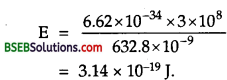For photons, using formula E = pc, we get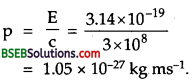(b) Let n = Number of photons emitted per second = No. of photons reaching the target/sec = ? ∴ Energy emitted by these photons/sec. = nhv = nE. Also P = energy emitted/sec = 9.42 x 10-3 W. ∴ nE = P or n = 𝑝𝐸 = 9.42×10−33.14×10−19 = 3 x 1016

(c) Let v = speed of hydrogen atom = ? m = mass of hydrogen atom = mass of proton = 1.67 x 10-27kg. Let p’ = momentum of hydrogen atom = mv ∴ As per statement p’ = p = momentum of photon = 1.05 x 10-27 kg ms-1, or mv = 1.05 x 10-27 ∴ v = 1.05×10−271.67×10−27 = 0.628 ms-1 = 0.63 ms-1

Question 5. The energy flux of sunlight reaching the surface of the earth is 1.388 x 103 W/m2. How many photons (nearly) per square metre are incident on the Earth per second ? Assume that the photons in the sunlight have an average wavelength of 550 nm. Answer: Here, λ = wavelength of photons in the sunlight = 550 nm = 550 x 10-9 m. ø = Energy flux = Energy of number of photons from sun light reaching earth per second per unit area = 1.388 x 103 W/m2 = 1.388 x 103 J s-1 m-2 …….(1) [∴ P = 𝐸𝑡 ∴ W = 1 Js-1] h = plank’s constant – 6.62 x 1034 Js. c = 3 x 108ms-1. Let n = number of photons incident on earth per sec per m2 = ? Let E’ = energy of each photon = hv = ℎ𝑐λ If E be the energy of all photons reaching the surface of earth per sec. per unit area, then E = nE’ = nhv = 𝑛ℎ𝑐λ ………….(2) ∴ From (1) and (2), we get nhc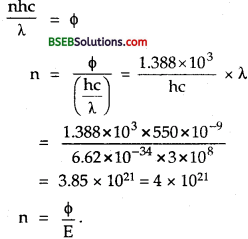Question 6. In an experiment on photoelectric effect, the slope of the cut-off voltage versus frequency of incident light is found to be 4.12 x 10-15 V s. Calculate the value of Planck’s constant. Answer: Here, slope of cut-off voltage versus frequency curve = tan θ = 𝑉𝑣 = 4.12 x 10-15Vs Where θ = angle made by the curve with the frequency axis, h = plank’s constant = ?, e = 1.6 x 10-19 C. Also we know thatQuestion 7. A100 W sodium lamp radiates energy uniformly in all directions. The lamp is located at the centre of a large sphere that absorbs all the sodium light which is incident on it. The wavelength of the sodium light is 589 nm. (a) What is the energy per photon associated with the sodium light? (b) At what rate are the photons delivered to the sphere? Answer: Here, P = power of sodium lamp = 100 W λ = wavelength of each photon = Wavelength of sodium light = 589 nm = 589 x 109 m. ∴Energy radiated per second by the lamp = 100 JS-1. (∴P = 𝐸𝑡) h = Plank’s constant = 6.62 x 10-34 JS, c = 3 x 108 ms-1.

(a) Energy per photon = E = ? Using formula, E = hv = ℎ𝑐λ, we get(b) Let n = rate at which the photons are delievered to the sphere = no. of photons delivered to sphere per sec = ?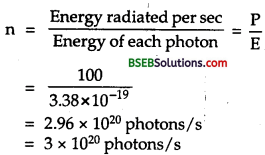Question 8. The threshold frequency for a certain metal is 3.3 x 1014 Hz. If light of frequency 8.2 x 1014 Hz is incident on the metal, predict the cut-off voltage for the photoelectric emission. Answer: Here, v0 = threshold frequency = 3.3 x 1014 Hz. v = frequency of incident light photons = 8.2 x 1014 Hz. V0 = cut-off voltage for photoelectric emission = ? h = 6.62 x 10-34 JS; e = 1.6 x 10-19 C. Using the relation, hv – hv0 = eV0, we get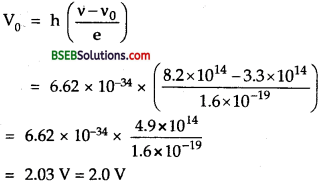Question 9. The work function for a certain metal is 4.2 eV. Will this metal give photoelectric emission for incident radiation of wavelength 330 nm? Answer: Here, W = Work function of certain metal = 4.2 eV = 4.2 x 1.6 x 10-19 J = 6.72 x 10-19 J. λ = Wavelength of incident radiation = 330 nm = 330 x 10-9 m. If E be the energy of the photon of incident light, then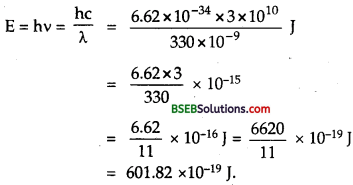As E < W, so photoelectric emission will not/take place. Aliter: Using relation hv0 = ω, we getAlso using formula, c = vλ, the frequency of incident radiations is given byNow from equation (1) and (2), it is clear that the frequency of incident radiation y is less than the threshold frequency y0, hence photoelectric emission for this radiation cannot take place.

Question 10. Light of frequency 7.21 x 1014 Hz is incident on a metal surface. Electrons with a maximum speed of 6.0 x 105 m/s are ejected from the surface. What is the threshold frequency for photoemission of electrons? Answer: Here, v’= frequency of incident light = 7.21 x 1014 Hz. υmax = maximum speed of electrons ejected from the surface = 6.0 x 105 ms-1. m = mass of electrons = 9.1 x 10-31 kg v0 = threshold frequency = ? h = Plank’s constant = 6.62 x 10-34 JS. Using Einstein’s photo electric equation, hv – hv0 = 12 mu2max , we get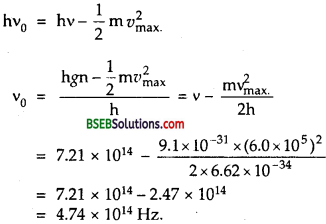Question 11. Light of wavelength 488 nm is produced by an argon laser which is used in the photoelectric effect. When light from this spectral line is incident on the emitter, the stopping (cut-off) potential of photoelectrons is 0.38 V. Find the work function of the material from which the cathode is made. Answer: Here, λ = wavelength of light = 488 nm = 488 x 10-9 m. V0 = Stopping potential = 0.38 V W = Work function of the material = ? c = 3 x 108 ms-1. h = Plank’s constant = 6.62 x 10-34 JS. e = 1.6 x 10-19 C. ∴Energy of incident photon = λv = ℎ𝑣λ = 6.62×10−34×3×108488×10−9 = 4.08 x 10-19 J. Using formula, eV0 = hv – W, we get W = hv – eV0 = 4.08 x 10-19J – 1.6 x 10-19 x 0.38 = (4.08 – 0.608) x 10-19 J = 3.472 x 10-19 J = 3.472×10−191.6×10−19 eV = 2.17 eV

Question 12. Calculate the (a) momentum, and (b) de-Broglie wavelength of the electrons accelerated through a potential difference of 56 V. Answer: Here, V = P.D. applied = 56 V

(a) p = momentum of electron = ? We know that 12 mυ2 = eV ∴ υ = 2eVm‾‾‾‾√, where m, υ are the mass and velocity of the electron. m = 9.1 x 10-31 kg, e = 1.6 x 10-19 C. Using p = mv, we get p = m = 2meV‾‾‾‾‾‾√ we get p = 2×9.1×10−31×1.6×10−19×56‾‾‾‾‾‾‾‾‾‾‾‾‾‾‾‾‾‾‾‾‾‾‾‾‾‾‾‾‾‾‾‾√ = 1.631×10−47‾‾‾‾‾‾‾‾‾‾‾‾‾√ or p = 4.04 x 10-24 kg ms-1,

(b) λ = de Broglie wavelength of the electron = ? λ = 12.27𝑉√ Å we get λ = 12.2756√ Å = 12.277.483Å = 1.64 Å = 1.64 x 10-10 m = 0.164 x 10-9m = 1.64 x 10 nm

Question 13. What is the (a) momentum. (b) speed, and (c) de Broglie wavelength of an electron with kinetic energy of 120 eV. Answer: Here, E = Kinetic energy of electron = 120 eV = 120 x 1.6 x 10-19 J = 1.92 x 10-17J m = mass of electron – 9.1 x 10-31 Kg

(a) h = 6.62 x 10-34 JS. momentum of electron = p = ? Using relation, E = p22 m, we get [∵E =12mv2=m2v22 m=p22 m] p = 22mE‾‾‾‾‾‾√ = 2×9.1×10−31×1.92×10−17‾‾‾‾‾‾‾‾‾‾‾‾‾‾‾‾‾‾‾‾‾‾‾‾‾‾‾‾‾√ = 5.91 x 10-24 kg ms-1,

(b) Let v = speed of electron = ? Using relation, p = mv, we get v = pm = 5.91×10−249.1×10−31 = 6.5 x 106 ms-1

(c) de-Broglie wavelength is given by λ = hp = 6.62×10−345.91×10−24 = 1.12 x 10-10 m = 0.112 x 10-9m = 0.112 nm

Question 14. The wavelength of light from the spectral emission line of sodium is 589 nm. Find the kinetic energy at which (a) an electron, and (b) a neutron, would have the same de- Broglie wavelength. Answer: Here, X = wavelength of light – 589 nm – 589 x 10-9 m. me= mass of electron = 9.1 x 10-31 kg. mn = mass of neutron = 1.67 x 10-27 kg. h = Plank’s constant = 6.62 x 10-34 JS.

(a) Let E1 = K.E. of electron = ? Using the relation λ = ℎ2𝑚𝑒𝐸√, we get λ2 = h22 meE(b) E2 = K.E of neutrons = ? it is calculated asQuestion 15. What is the de Broglie wavelength of (a) a bullet of mass 0.040 kg travelling at the speed of 1.0 km/s. (b) a ball of mass 0.060 kg moving at a speed of 1.0 m/s, and (c) a dust particle of mass 1.0 x 10-9 kg drifting with a speed of 2.2 m/s? Answer: (a) Here, mb = mass of bullet = 0.040 kg vb = velocity of bullet = 1.0 km s-1 = 1000 ms-1, h = 6.62 x 10-34 JS. λb = de Broglie wavelength of bullet = ? Using formula, λ = hmv, we get λb = hmb𝑣b=6.62×10−340.040×1000 = 1.655 x 10-35 m = 1.7 x 10-35 m

(b) Here, m = 0.060 kg v = 1.00 ms-1 λ = ? ∴ λ = hmv=6.62×10−3460×10−3×1.0 = 1.1 x 10-32 m

(c) Here, m = 1.0 x 10-9 kg v = drift speed of dust particle = 2.2ms-1 λ = deBroglie wavelength = ? p = mv =10 x 10-9 x 2.2 = 2.2 x 10-9 kg ms-1 λ = ℎ𝑚𝑣 = ℎ𝑝 = 6.62×10−342.2×10−9 = 3.01 x 10-25 m.

Question 16. An electron and a photon each have a wavelength of 1.00 nm. Find (a) their momenta (b) the energy of the photon, and (c) the kinetic energy of electron. Answer : Here λc = wavelength of an electron = 1.0 nm = 10-9 m. λp = wavelength of a photon = 10-9 m. h = 6.63 x 10-34Js, c = 3 x 108 ms-1

(a) (i)Momentum of electron = pe Using formula, λ = ℎ𝑝, we get

(b) Let Ep = Kinetic energy of photon = ? pe = h𝜆e = 6.63×10−3410−9 = 6.63 x 10-25 kg ms-1

(ii) Let pp = momentum of photon ∴ pp = ℎ𝜆𝑝=6.63×10−3410−9 = 6.63 x 10-25 kg ms-1

(b) Let Ep = kinetic enegry of photon = ? Using formula, E = hv . ℎ𝑐λ , we get(c) Ee = K.E of electron Using formula,Question 17. (a) For what kinetic energy of a neutron will the associated de Broglie wavelength be 1.40 x 10-10 m? (b) Also find the de Broglie wavelength of a neutron, in thermal equilibrium with matter, having an average kinetic energy of 32 k T at 300 K. Answer: (a) Here, λ = deBroglie wavelength = 1.40 x 10-10 m. h = 6.62 x 10-34 Js, E = Kinetic energy of neutron = ? m = mass of neutron = 1.67 x 10-27kg.

Using formula, λ = h2mE√, we get(b) Here, T = absolute Temperature = 300 K k = Boltzman’s constant = 1.38 x 10-23 JK-1. E = Kinetic energy of neutron = 32 kT = 32 x 1.38 x 10-23 x 300 = 6.21 x 10-21 J. m = mass of neutron = 1.67 x 10-27 kg. λ = deBroglie wavelength of neutron = ?

Using the relation,Question 18. Show that the wave length of electromagnetic radiation is equal to the de Brogue wave length of its quantum. Answer: Let v, λ’ be the frequency and wave length of the photon having momentum ∴ p = EC=hvC Where C = speed of photon = Speed of electromagnetic radiation ∴ de Brogue wavelength A’ of the photon i.e. quantum of e.m. wave is given by λ’ = hp=h(hvC) = hh2=Cv ……….(1) Also let A be the wavelength of electromagnetic radiation of frequency V. ∴ 𝜆=𝐶𝑣 ……….(2) where C = speed of photon = speed of electromagnetic radiation. ∴ From (1) and (2) λ = λ’ = 𝐶λ Hence Proved.

Question 19. What is the de Brogue wavelength of a nitrogen molecule in air at 300 K? Assume that the molecule is moving with the root-mean-square speed of molecules at this temperature. (Atonlic mass of nitrogen = 14.0076 u) Answer: Here, k BoltzmaWs constant = 1.38 x 10-23 JK-1. T = absolute temp = 300 K m = moLecular weight of Nitrogen molecule = 28.0152 u = 28.0152 x 1.67 x 10-27 Kg Let C = r.m.s. speed of N2 molecule at 300 K. ∴ According to Kinetic Theory of gases, K.E./molecule = 32 kT or C = 3kTm‾‾‾‾√ C = 3×1.38×10−23×30028.0152×1.67×10−27‾‾‾‾‾‾‾‾‾‾‾‾‾‾‾√ = 5.15 x 102 ms-1 λ = deBroglie wavelength = ?

Using formula, λ = hmC = 6.62×10−3428.0152×1.67×10−27×5.15×102 = 2.75 x 1011 m = 0.0275 x 109 m = 0.0275 nm. = 0.028 nm

Question 20. (a) Estimate the speed with which electrons emitted from a heated emitter of an evacuated tube impinge on the collector maintained at a potential difference of 500 V with respect to the emitter. Ignore the small initial speeds of the electrons. The ‘specific charge’ of the electron i.e., its – is given to be 1.76 x 1011 C kg-1.

(b) Use the same formula you employ in (a) to obtain electron speed for a collector potential of 10 MV. Do you see what is wrong? In what way is the formula to be modified? Answer: (a) Here, V = potential difference between cathode and anode = 500 V. em = specific charge of electron = 1.76 x 1011 C kg-1. υ = speed of emission of electrons from cathode = ? Using the relation, eV = 12 mv2 …………..(1) we get υ = 2emV‾‾‾‾‾√ ……….(2) or υ = 2×1.76×1011×500‾‾‾‾‾‾‾‾‾‾‾‾‾‾‾‾‾‾‾‾√ = 1.33 x 107 ms-1,

(b) Here, V = anode potential = 10 MV = 10 x 106 V = 107 V. Using equation (2), υ = 2𝑒𝑚𝑉‾‾‾‾√ υ = 2×1.76×1011×107‾‾‾‾‾‾‾‾‾‾‾‾‾‾‾‾‾‾‾‾√ = 1.88 x 109 ms-1 This velocity υ is greater than the velocity of light C in free space (which is 3 x 108 ms-1). No material particle can have velocity comparable to C. Therefore, K.E. = 12 mv2 is not exact relation. So, the formula of K.E. is to be modified by taking into consideration special theory of relativity. Thus the relativistic expression for K.E. replacing 12 mv2 becomes mc2 – mQ c2 = c2 (m – m0) where m0 is the rest mass of an electron = 9 x 10-31 kg and m is relativistic mass of electron given by m = 𝑚01−𝑣2𝐶2√ ∴ eqn. (1) is to be modified as : (m – m0) c2 = eV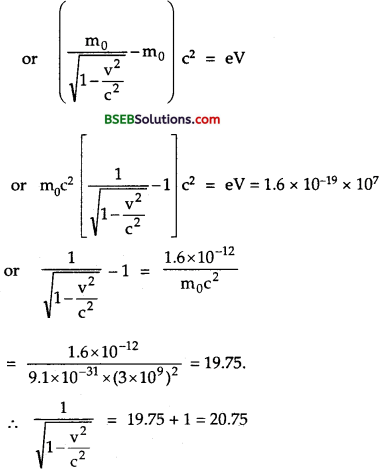Squaring on both sides, we get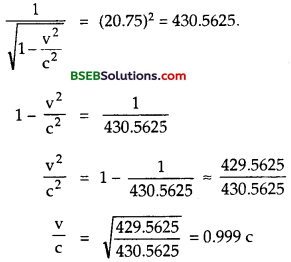i. e. v is now comparable to c.

Question 21. (a) A monoenergetic electron beam with electron speed of 5.20 x 106 ms-1 is subject to a magnetic field of 1.30 x 10-4 T normal to the beam velocity. What is the radius of the circle traced by the beam, given – for electron equals 1.76 x 1011 C kg-1.

(b) Is the formula you employ in (a) valid for calculating radius of the path of a 20 MeV electron beam? If not, in what way is it modified? [Note : Exercises 11.20 (b) and 11.21 (b) take you to relativistic mechanics which is beyond the scope of this book. They have beer, inserted here simply to emphasise the point that the formulas you use in part (a) of the exercises are not valid at very high speeds or energies. See answer at the end to know what ‘very high speed or energy means.] Answer: Here, υ = speed of electrons beam = 5.20 x 106 ms-1. B = magnetic field = 1.30 x 10-4 T 𝑒𝑚 = specific charge of electron = 1.76 x 1011 C kg-1. m θ = angle between 𝐵⃗ and the υ⃗ = 90° Force exerted by 𝐵⃗ on the electron is 𝐹¯𝑚 = e(𝑣⃗ ×𝐵⃗ ) Fm = e υ B sin 90° = eυB This force provides the necessary centrepetal force for tracing out a circle i.e.,(b) Here, E = energy = 20 MeV= 20 x 1.6 x 10-13J Using relation, E = 12 mv2, we get υ = 2Em‾‾‾√=2×20×1.6×10−139.1×10−31‾‾‾‾‾‾‾‾‾‾‾‾√ = 2.67 x 109 ms-1 which is greater than the velocity of light. So the formula used in case (a) i.e., r = mveB is not valid for calculating the radius of the path of 20 MeV electron beam because electron with such a high energy has velocity in the relativistic domain i.e., comparable with the velocity of light and the mass varies with the increase in velocity but we have taken it as constant. ∴m = 𝑚01−𝑣2𝑐2√ is to be considered. Thus the modified equation becomes : r = ⎛⎝⎜⎜𝑚01−𝑣2𝑐2√⎞⎠⎟⎟𝑣𝑒𝐵

Question 22. An electron gun with its collector at a potential of 100 V fires out electrons in a spherical bulb containing hydrogen gas at low pressure (~ 10-2mm of Hg). A magnetic field of 2.83 x 10-4 T curves the path of the electrons in a circular orbit of radius 12.0 cm. (The path can be viewed because the gas ions in the path focus the beam by attracting electrons, and emitting light by electron capture : this method is known as the ‘fine beam tube’ method.) Determine e/m from the data. Answer: Here, V = potential at anode = 100 V B = magnetic field = 2.83 x 10-4 T radius of circular path, r = 12 cm = 0.12 m 𝑒𝑚 = ? Let υ = Velocity acquired by electrons m = mass of electron ∴Gain in K.E. of electrons when accelerated through V volts is given by 12mv2 = eV or mv2 = 2eV As the electrons move in the circular path so magnetic force = Centrepetal force or eυB = mv2r or v = eBrm ………..(ii) ∴ From (i) and (ii), we get m (eBrm)2 = 2eV or 𝑒𝑚 = 2 V B2r2 = 2×100(2.83×10−4)2×(0.12)2 = 1.73 x 1011 C Kg1

Question 23. (a) An X-ray tube produces a continuous spectrum of radiation with its short wavelength end at 0.45 A. What is the maximum energy of a photon in the radiation? (b) From your answer to (a), guess what order of accelerating voltage (for electrons) is required in such a tube? Answer: Here, λ min = 0.45 A = 0.45 x 1010 m. Emax = maximum energy of X-ray photon = ? h = Plank’s constant = 6.62 x 10-34 JS. c = Velocity of light = 3 x 108 ms-1.

Using Formula,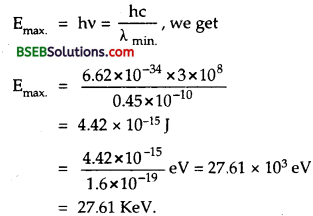(b) In X-ray tube, accelerating voltage provides the energy to the electrons which produce X-rays. For getting X-ray photons of 27.61 KeV, it is required that the incident electrons must possess kinetic energy of atleast 27.61 KeV. The order of the accelerating voltage can be calculated as : Emax = eV V = 𝐸max𝑒=4.42×10−51.6×10−19 V = 27.61 x 103 V = 27.61 KV. Order of the accelerating voltage is 30 KV.

Question 24. In an accelerator experiment on high-energy collisions of electrons with positrons, a certain event is interpreted as annihilation of an electron-positron pair of total energy 10.2 BeV into two γ-rays of equal energy. What is the wavelength associated with each γ-ray? (1 BeV = 109 eV) Answer: Here, Energy of 2γ-rays = 10.2 BeV = 10.2 x 109 eV (∴1 BeV = 109 eV). ∴ Energy of each y-ray is given by E = 12 (10.2 x 109 eV) = 12 x 10.2 x 109 x 1.6 x 10-19 J = 8.16 x 10-10 J. λ = wavelength of each γ-ray = ? Using formula, E = ℎ𝑐λ, we get λ = ℎ𝑐𝐸 = 6.62×10−34×3×1088.16×10−10 = 2.44 x 10-16 m.

Question 25. Estimating the following two numbers should be interesting. The first number will tell you why radio engineers do not need to worry much about photons! The second number tells you why our eye can never ‘count photons’, even in barely detectable light. (a) The number of photons emitted per second by a Medium wave transmitter of 10 kW power, emitting radiowaves of wavelength 500 m. (b) The number of photons entering the pupil of our eye per second corresponding to the minimum intensity of white light that we humans can perceive (~10-10 Wm-2). Take the area of the pupil to be about 0.4 cm2, and the average frequency of white light to be about 6 x 1014 Hz. Answer: (a) Here, P = power of transmitter = 10 kVV = 104 W λ = wavelength of radiowaves = 500 m. h = 6.626 x 10-34 JS, c = 3 x 108 ms-1. Let E = energy of each photon ∴ E = hv = ℎ𝑐λ = 6.626×10−34×3×108500 = 3.98 x 10-28 J n = no. of photons emitted per second = ? n is given byWe see that the energy of a radio photon is very small and the number of photons emitted per second in a radio beam is very large. So there is negligible error involved in ignoring the energy of photon and we can treat total energy of radio waves as continuous.

(b) Here, A = area of pupil = 0.4 cm2 = 0.4 x 10-4 m2. v = average frequency of white light = 6 x 1014Hz I = minimum intensity of white light = 10-10Wm-2. ∴ E = energy of each photon = hv = 6.626 x 10-34 x 6 x 10-14 = 3.98 x 10-19 J. Let n = no. of photons falling per sec. per unit area. ∴ energy per unit area per sec. due to these photons. = total enrgy of n photons. = nE = n x 3.98 x 10-19 Js-1m-2 Also I = energy per sec. per unit area. ∴ 10-10 = n x 3.98 x 10-19 or n = 10−113.98×10−14 = 2.51 x 108 m-2s-1 ∴ No. of photons entering the pupil per second n x area of pupil = 2.51 x 108 x 0.4 x 10-4 ≈ 104 s-4. Though this number is not as large as in (a). but still large enough to be cou nted Comparison of case (a) and (b) tells that our eye cannot count the number of photons individually.

Question 26. Ultraviolet light of wavelength 2271 A from a 100 W mercury source irradiates a photo-cell made of molybdenum metal. If the stopping potential is – 1.3 V, estimate the work function of the metal. How would the photo-cell respond to a high intensity (~ 10-5 W m-2) red light of wavelength 6328 Å produced by a He-Ne laser? Answer: Here, Power P = 100 W Stopping potential, V0 = 1.3 V (magnitude) λr = wavelength of red light = 6328 Å = 6328 x 10-10 m. h “ plank’s constant – 6.62 x 108ms-1 Js, c = 3 x 108 ms-1. h = wavelength of UV light = 2271 A = 2271 x 10-10 m. λu = work function of metal = ? Using Einstein’s photoelectric equation,Let v0 = frequency for red light. ∴ hv0 = W or v0 = Wh=4.17×1.6×10−196.62×10−34 = 1.0 x 1015 Hz Let vr = frequency for red light. vr = 𝑐𝜆𝑟 = 3×1086328×10−10 = 4.74 x 1014Hz Since vr < v0 i.e., frequency produced by He-Ne laser incident on the photocell is lesser than the threshold frequency, so the photocell will not respond.

Question 27. Monochromatic radiation of wavelength 640.2 nm (1 nm = 109 m) from a neon lamp irradiates photosensitive material • made of caesium on tungsten. The stopping voltage is measured to be 0.54 V. The source is replaced by an iron source and its 427.2 nm line irradiates the same photo-cell. Predict the new stopping voltage. Answer: Here, for neon lamp; λ = wavelength = 640.2 nm = 640.2 x 10-9 m. V0 = Stopping potential = 0.54 V. h = 6.62 x 1034 Js, c = 3 x 108 ms-1 . W = Work function = ? Using formula,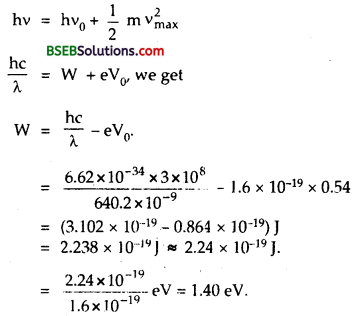For iron source : λ = wavelength = 427.2 nm = 427.2 x 10-9 m W = work function = 2.238 x 10-19J V0 = stopping potential = ? From eqn. (1), we get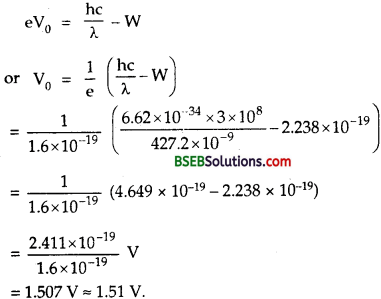Question 28. A mercury lamp is a convenient source for studying frequency dependence of photoelectric emission, since it gives a number of spectral lines ranging from the UV to the red end of the visible spectrum. In our experiment with rubidium photo-cell, the following lines from a mercury source were used : λ1 = 3650 Å, λ2 = 4047 Å, λ3 = 4358 Å, λ4= 5461 Å, λ5= 6907 Å, The stopping voltages, respectively, were measured to be : V01 = 1.28 V, V02 = 0.95 V, V03 = 0.74 V, V04 = 0.16 V, V05 = 0V (a) Determine the value of Planck’s constant h the threshold frequency and work function for the material. [Note : You will notice that to get h from the data, you will need to know e (which you can take to be 1.6 x 10 19 C). Experiments of this kind on Na, Li, K etc. were performed by Milikan, who, using his own value of e (from the oil-drop experiment) confirmed Einstein’s photoelectric equation and at the same time gave an independent estimate of the value of h ] Answer: From Einstein photoelectric equation, eV0 = hv – W or hv – eV0 = W or ℎ𝑐λ – eV0 = W ………(1) For λ = λ1, V0 = V01, we get hc𝜆1 – eV01 = W ………..(2) and for λ = λ2, V0 = V02, we get hc𝜆2 – eV02 = W …………(3) (2) – (3) gives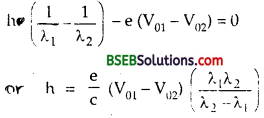Using e = 1.6 x 10-19C, c = 3 x 108 ms-1 and values of λ1, λ2and V01 V02from table, we get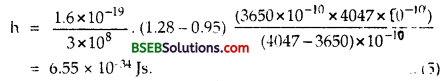Similarly taking the set of values λ2, λ3 V02 and V03 We getSimilarly taking the set of Values, λ3, λ03and V04, we get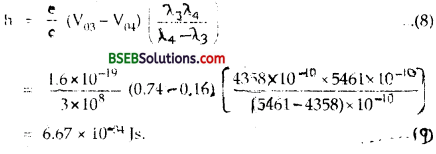From (5), (7) and (9), we conclude that the approximate value of h is 6.6 x 10-34 Js. From eqn. (2), we getQuestion 29. The work function for the following metals is given : Na : 2.75 eV; K : 2.30 eV; Mo : 4.17 eV; Ni : 5.15 eV. Which of these metals will not give photoelectric emission for a radiation of wavelength 3300 A from a He-Cd laser placed 1 m away from the photocell? What happens if the laser is brought nearer and placed 50 cm away? Answer: Here, for all metals wave length of incident radiation, λ = 3300 Å = 3300 x 10-10 m. ∴ Frequency of incident radiation is given by : v = 𝑐λ = 3×1083300×10−10 = 9.1 x 1014Hz If v0 be the threshold frequency, then hv0 = W v0 = Wh ……….(1) Also it is given that∴ Threshold frequencies for various metals are :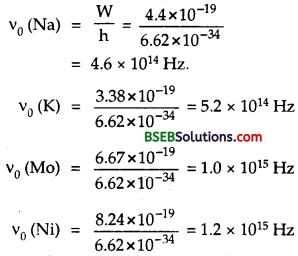Now we see that the frequency of incident radiation is greater than the threshold frequency of Na and K metals, but lesser than those of Mo and Ni. So thephotoelectric emission will take place only in Na and K metals and not in Mo and Ni. Alliter : Energy of incident radiation is –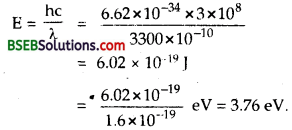which is greater than W of Na and K and lesser than that for Mo and Ni. So emission in Na and K will take place. Now r = 1 m, r’ = 50 cm = 0.50 m. If the laser is brought closer, the intensity of incident radiation increases. This does not affect the result regarding Mo and Ni metals, while photoelectric emission from Na and K will increase in proportion to intensity as I ∝ 1𝑟2 and becomes 4 times when r’ = 𝑟2

Question 30. Light of intensity 10-5 W m-2 falls on a sodium photo-cell of surface area 2 cm2. Assuming that the top 5 layers of sodium absorb the incident energy, estimate time required for photoelectric emission in the wave-picture of radiation. The work function for the metal is given to be about 2 eV. What is the implication of your answer? Answer: Here; I = intensity of incident light = 105 Wm-2. A = surface area of each layer in sodium = 2 cm2 = 2 x 10-4 m2. W = Work function for metal = 2eV = 2 x 1.6 x 10-19 J. Since r = size of atom is about 10-10 m (= 1 Å), so the effective area of an atom of Na may be considered as ≈ r2 = 10-20 m2 (roughly) ∴ Number of atoms in the 5 layers of sodium =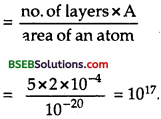We know that sodium has one free electron (or conduction electron) per atom. ∴ No. of electrons in 5 layers of sodium = 1017. Now the incident power on the surface area of the photocell, P = IA = 102 x 2 x 10-4 = 2 x 10-9 W.

Since incident energy is absorbed by 5 layers of sodium, so according to wave picture of radiation, the electron present in all the 5 layers of sodium will share the incident energy equally. ∴ energy recieved by any one electron in 5 layers of sodium =Since the work function of sodium is 2 eV, an electron w.ill be ejected as soon as it gathers energy’ equal to 2eV. ∴ time required for photoelectric emission 𝑊𝐸=3.2×10−192×10−26 = 1.6 x 107s ≈ 0.5years Implication : The answer obtained implies that the time of emission of electron is very large and is not in agreement with the observed time of emission, which is nearly 10″9 s i.c., there is no time lag between the incidence of light and the emission of photo electrons. Thus we conclude that wave-picture of radiation is not applicable for photo-electric emission.

Question 31. Crystal diffraction experiments can be performed using X-rays, or electrons accelerated through appropriate voltage. Which probe has greater energy? (For quantitative comparison, take the wavelength of the probe equal to lA, which is of the order of interatomic spacing in the lattice) (me = 9.11 x 10-31 kg). Answer: Here, λ = wavelength of the probe = 1 Å = 10-10m. me = mass of electron = 9.11 x 10-31 kg. h = Plank’s constant = 6.62 x 10-34 JS. c = 3 x 108 ms-1 For X-ray photon of 1Å – If Ex be the energy of X-ray photon, then Using formula, E = ℎ𝑐λ, we getFor electron of wavelength 1Å Using formula, λ = ℎ𝑚υ, we get mυ = ℎ𝜆=6.62×10−3410−10 = 6.62 x 10-24 kg ms-1 if E be the enegry of electron, thenClearly X-ray photon has much greater energy than energy of electron for same wavelength.

Question 32. (a) Obtain the de Broglie wavelength of a neutron of kinetic energy 150 eV. As you have seen in Exercise 11.31, an electron beam of this energy is suitable for crystal diffraction experiments. Would a neutron beam of the same energy be equally suitable? Explain. (mn = 1.675 x 10-27 kg). (b) Obtain the de Broglie wavelength associated with thermal neutrons at room temperature (27 °C). Hence explain why a fast neutron beam needs to be thermalised with the environment before it can be used for neutron diffraction experiments. Answer: (a) Here, mn = mass of neutron = 1.675 x 10-27 kg En = Kinetic energy of neutron = 150 eV = 150 x 1.6 x 10-19 J = 2.4 x 10-17 J. λn = wavelength of neutron = ? Using Formula,This wavelength is about hundred times smaller than the interatomic spacing (≈ 10-10 m) of the crystals, thus a neutron of 150 eV energy is not suitable for crystal diffraction experiments. (b) Here, T = 27°C = 273 + 27 = 300 K mn = 1.675 x 10-27 kg, k = 1.38 x 10-23J k-1 molecule-1 E = energy of neutron at temperature T = 32 kT λ = wavelength of thermal neutrons = ? Using formula,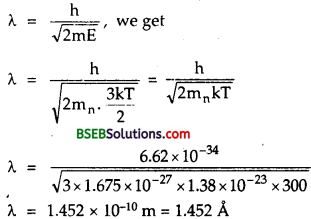This wavelength is comparable to the interatomic spacing of crystals (≈ 10-10 m). So thermal neutrons are able to interact with the crystal and hence are suitable for diffraction experiments. Since λ ∝ 1T√, so increasing their temperature, decreases their de Broglie wavelength and they become unsuitable for crystal diffraction. Fast neutrons possess wavelength quite small as compared to interatomic spacing. Hence they need to be thermalised with the environment for neutron diffraction experiments.

Question 33. An electron microscope uses electrons accelerated by a voltage of 50 kV. Determine the de Broglie wavelength associated with the electrons. If other factors (such as numerical aperture, etc.) are taken to be roughly the same, how does the resolving power of an electron microscope compare with that of an optical microscope which uses yellow light? Answer: Here, V = acceleration potential = 50 KV = 50 x 103 V. m = 9.1 x 10-31 kg, e = 1.6 x 10-19 C, h = 6.62 x 10-34 Js. λe = wavelength of electron = ? E = energy of electrons is given by E = 12 mv2 = eV or E = eV = 1.6 x 10-19 x 50 x 103J = 80 x 10-16 J

Using formula, λ = h2mE√, we get , λe = h2mE√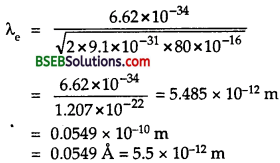Also for yellow light λy = 5990 Å x 10-10m. Also we know that the resolving power of a microscope is inversely proportional to the wavelength of the radiation used. i.e R.P ∝1λi.e R.P. of elecron Microscope is 105 times larger than the R.P of optical Microscope

Question 34. The wavelength of a probe is roughly a measure of the size of a structure that it can probe in some detail. The quark structure of protons and neutrons appears at the minute length-scale of 10 15 m or less. This structure was first probed in early 1970’s using high energy electron beams produced by a linear accelerator at Stanford, USA. Guess what might have been the order of energy of these electron beams. (Rest mass energy of electron = 0.511 MeV.) Answer: Here, λ = size of structure = 10-15 m. h = 6.62 x 10-34 Js, c = 3 x 108 ms-1. m0 C2 = rest mass energy = 0.511 MeV = 0.511 x 1.6 x 10-13 J = 8.18 x 10-14 J. ∴ Using the relation, λ = ℎ𝑝, we get P = ℎλ = 6.62×10−3410−15 = 6.62 x 10-19 kg ms-1. Using the relativistic formula for total energy of particle, we get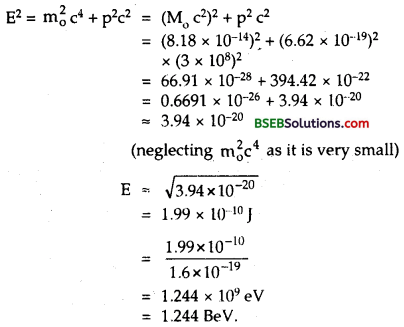Thus energy of the proton ejected out of the linear accelerator is of the order of BeV.

Question 35. Find the typical de Broglie wavelength associated with a He atom in helium gas at room temperature (27°C) and l atm pressure; and compare it with the mean separation between two atoms under these conditions. Here, t = 27°C Atomic mass of He = 4g T = 273 + 27 = 300 k N = Avogadro’s number h = 6.62 x 10-34sec. = 6 x 1023 Let m = mass of He atom∴ Using the relation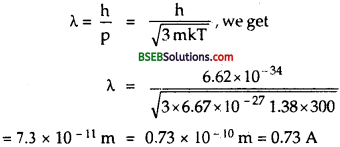Let r0 be the mean separation between the two atom = ? P = 1 atom = 1.01 x 105 Pascal/m2 Using the relation, PV = RT + b NT (∴ R = kN) ∴ VN=𝑘 TP ∴Mean separation between two atom is given by : r0 = (vN)1/3From (1) and (2), we see that so is much logner than de Broglie wave length. i.e 𝜆r0=0.73×10−1034×10−10 = 0.021 m

Question 36. Compute the typical de Broglie wavelength of an electron in a metal at 27°C and compare it with the mean separation between two electrons in a metal which is given to be about 2 x 10-10 m. [Note : Exercises 11.35 and 11.36 reveal that while the wave- packets associated with gaseous molecules under ordinary conditions are non-overlapping, the electron wave-packets in a metal strongly overlap with one another. This suggests that whereas molecules in an ordinary gas can be distinguished apart, electrons in a metal cannot be distinguished apart from one another. This indistinguishibility has many fundamental implications which you will explore in more advanced Physics courses.] Answer: Here t = 27° C ∴ T = 27 + 273 = 300 K. M = mass of an electron = 9.1 x 10-31 kg λ = de Broglie wavelength = ? λ = 6.62 x 10-34sec k = Boltzmann’s contant = 1.38 x 10-23JK-1 mol-1 r0 = mean separation between two electrons in a metal = 2 x 10-10 m = 2 A. K.E. of electOm at T is given by E = 32 KT. de Broglie wave length of electron is given byi.e. λ is 31 times the inter-electron separation (r0) in a metal. Thus from Q. 35 and Q. 36, we conclude that electron wave packets. In a metal strongly overlap with one another while the wave packets associated with gaseous molecules under ordinary conditions don’t overlap.

Question 37. Answer the following questions : (a) Quarks inside protons and neutrons are thought to carry fractional charges [(+2/3)e ; (-1/3)e]. Why do they not show up in Millikan’s oil-drop experiment ? Answer: (a) The quarks having fractional charges are thought to be confined within a proton and a neutron. These quarks are bound by forces. These forces become stronger when the quarks are tried to be pulled a part. That is why, the quarks always remain together. It is due to this reason that though fractional charges does not exist in nature but the observable charges are always integral multiple of charge of electrons.

(b) What is so special about the combination 𝑒𝑚 not simply talk of e and m separately? Answer: Both the basic relations eV = 12 mV2 or eE = ma and eBv = mV2r for electric and magnetic fields respectively show that the dynamics of electrons is determined not by and m separately but by the combination of 𝑒𝑚

(c) Why should gases be insulatprs at ordinary pressures and start conducting at very low pressures? Answer: At low pressure, ions have a chance to reach their respective electrodes and constitute a current. At ordinary pressures, ions have no chance to db so because of collisions with gas molecules and recombination.

(d) Every metal has a definite work function. Why do all ’ photoelectrons not come out with the same energy if incident radiation is monochromatic? Why is there an energy distribution of photoelectrons? Answer: Work function merely indicates the minimum energy required for the electron in the highest level of conduction band to get out of the metal. Not all electrons in the metal belong to this level. Consequently, for the same incident radiation, electron knocked off from different levels come out with different energies.

(e) The energy and momentum of an electron are related to the frequency and wavelength of the associated matter wave by the radiations : E = hv, p = ℎλ But while the value of A is physically significant, the value of v(and therefore, the value of the phase speed vλ) has no physical significance. Why? Answer: The absolute value of energy E (but not momentum) of any particle is arbitrary to within an additive constant. Hence, while λ is physically significant, absolute value of v of matter wave of an electron has no direct physical meaning. The phase speed vλ is likewise not physically significant. The group speed given by 𝑑𝑣𝑑(1𝜆)=𝑑𝐸𝑑𝑝 = 𝑑𝑑𝑝(𝑝22𝑚)=𝑝𝑚 is physically meaningful.

### Bihar Board Class 12 Physics Dual Nature of Radiation and Matter Additional Important Questions and Answers

Question 1. An electron and a proton are projected in an electric field at right angles to the field with same velocity. Which particle has more curved trajectory? Answer: We know that the transverse deflection of the particle in E is given by As the mass of electron is lesser than the mass of proton, so the transverse deflection of electron is more than that of the proton. Hence the trajectory of the electron will be more curved than that of the proton.

Question 2. Why bigger oil drops are not used in Millikan’s experiment? Answer: If bigger oil drops are used then very strong electric field will be required to conduct the experiment which may not be practically possible.

Question 3. How did Millikan’s experiment lead to the quantum nature of electrical charge? Answer: It is found that the oil drop has always a charge which is the integral multiple of a basic charge i.e., e having value given by e = 1.6 x 10-19 C.

Question 4. Define work function of a metal. Answer: It is defined as the minimum amount of energy required by electrons to leave the surface of the metal.

Question 5. Define 1 eV. Answer: It is defined as the kinetic energy acquired by the free electron while passing through a potential difference of 1 volt. i.e. leV = 1.6 x 10-19.

Question 6. Which photon out of the red or violet one is more energetic? Why? Answer: Violet photon is more energetic. We know that the energy of a photon is given by E = hv = ℎ𝑐λ Also we know that λv < λr, Thus as E is inversely proportional to the wavelength, so Ev > Er.

Question 7. Why alkali metals are most suitable for photoelectric effect? Answer: We know that the work function of alkali metal is small, so even the radiations of small energy are able to cause photoelectric emission.

Question 8. Out of copper and sodium which one has greater work function and threshold wavelength? Answer: The work function of copper is greater than that of sodium. As W = hv0 = hc𝜆0 and Wc> Ws, so the threshold wavelength for sodium . is greater than that of copper.

Question 9. Define a photon. What is its rest mass and velocity? Answer: It is defined as the quanta or packet of light energy. Its rest mass (mQ) is zero and velocity is 3 x 108 ms-1.

Question 10. Define a photo cell. Answer: It is defined as a device which converts light energy into electrical energy.

Question 11. Name the phenomenon which illustrates the particle nature of light. Answer: Photo electric effect illustrates the particle nature of light.

Question 12. Can all metals emit photo electrons when exposed to visible light? Name them. Answer: No, all metals cannot emit photo electrons when exposed to visible light. Only alkali metals can emit photo electrons when exposed to visible light.

Question 13. Define stopping potential. Answer: It is defined as the value of minimum negative potential applied to the plate of a photocell at which photo electric current becomes zero.

Question 14. Define cut off or threshold frequency. Answer: It is defined as the minimum frequency of photon to cause photoelectric emission.

Question 15. Name the experiment which verified the wave nature of particles. Answer: Davisson and Germer experiment verified the wave nature of particles.

Question 16. Is de-Broglie wavelength of a photon of electromagnetic wave equal to the wave length of the electromagnetic wave? Answer: Yes/de-Broglie wavelength of a photon of electromagnetic wave is equal to the wave length of the e.m. wave.

Question 17. Define a matter wave or a de-Broglie wave. Answer: It is defined as a wave associated with a material particle.

Question 18. Why are de-Broglie waves not electromagnetic in nature? Answer: Electromagnetic waves are produced only by the accelerated charged particle. But de-Broglie waves are always associated with every moving material particle (whether charged or uncharged). So de-Broglie waves are not electromagnetic in nature. ‘

Question 19. The de-Broglie wavelength of a particle of mass m moving with a velocity v is : λ = 𝐡mv What will be the modification in X when velocity of the particle is comparable to the velocity of light? Answer: When the particle moves with the velocity v which is comparable to the velocity of light, then the mass m of the particle change as : m = 𝑚𝑜1−𝑣2𝑐2√ Thus modified wavelength becomes : λ = ℎ𝑚𝑣=ℎ𝑚0𝑣1−𝑣2𝑐2‾‾‾‾‾‾√

Question 20. If the frequency of the incident radiation of the cathode of a photocell is doubled, how the following change? (a) photoelectric current. (b) stopping potential. (c) kinetic energy of the electrons. Answer: (a) If the frequency of incident radiation is doubled, there will be no change in the number of photo electrons i.e., photo electric current. (b) We know that hv = hv0 + eV0 or eV0 = hv – hv0 …………(1) Thus as frequency is doubled the difference hv – hv0 increases, so V0 (stopping potential) increases as V0 ∝ v, h, V0 being constants. (c) We know that hv = hv0 + 12 mv2max or 12 mv2max = hv – hv0 as h , v0 are constants ∴ K.E ∝ v Thus when v is doubled hv – hvQ difference increases, hence K.E. increases.

Question 21. What is the acceleration of an electron in the electric field of magnitude 100 Vcm-1, 𝑒𝑚 = 1.76 x 1011C Kg-1 Answer: Here, E = 100 Vcm-1 = 10000 Vm-1 𝑒𝑚 = specific charge of the electron = 1.76 x 1011 C kg-1. Let a = acceleration of electron in the electric field = ? Using the relation, a = 𝑒𝐸𝑚 = 𝑒𝑚 E, we get a = 1.76 x 1011 x 10000 = 1.76 x 1015 ms-1.

Question 22. Define specific charge of an electron. Give its S.I. unit and value for an electron. Answer: It is defined as the ratio of charge to mass of an electron. Its SI unit is C kg-1 and its value for electron is given by 𝑒𝑚 = 1.76 x 1011 C kg-1.

Question 23. On what factors does the threshold frequency depends? Does it depends upons the intensity of light? Answer: It depends upon the nature of material only emitting photo-electrons. No, it does not depend upon the intensity of light.

Question 24. A photon and electron have got same de-Broglie wavelength (≈ 10-10 m). Which has greater K.E.? Explain. Answer: We know that energy of photon is given by E1 = ℎ𝑐λ ………….(1) Also if E2 be the energy associated with the electron, then using formula,or 𝐸1𝐸2=901.1 > 1, ∴E1 > E2. Thus photon has more K.E. than electron.

Question 25. An electron and a proton have the same amount of K.E. which of the two have greater de-Broglie wavelength? Explain. Answer: Let λe and λp be the de-Broglie wavelength of the electron and a proton respectively, Ee and Ep are tneir K.E., Ee = Ep. ∴Using formula, λ = ℎ2𝑚𝐸√, we get λe = ℎ2𝑚𝑒𝐸𝑒√ and λp = ℎ2𝑚𝐹𝐸𝑝√ ∴ 𝜆e𝜆2p = 𝐸𝑝𝑚𝑝𝐸𝑒𝑚𝑒‾‾‾‾‾√=𝑚𝑝𝑚𝑒‾‾‾√ (∴ Ee = Ep)

Now as mpme > 1, ∴Wavelength associated with electron is more.

Question 26. A proton and an electron have same dc-Brogue wavelength. Which of them will move faster? Why? Answer: Electron moves faster than proton. We know that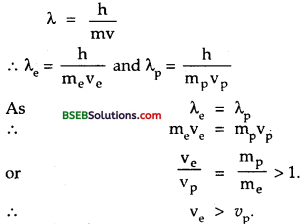Question 27. λe = λpWhich of the two have greater K.E.? Answer: We know thati.e. Kinetic energy of electron is greater than the K.E. of proton.

Question 28. What determines the maximum velocity of the photoelectrons? Answer: The work function of the metallic surface and the frequency of the incident radiations determine the maximum velocity of the photo electrons.

Question 29. Is there any difference between Light waves and matter waves? Answer: Yes, the velocity of tight waves in vacuum is constant whereas the velocity of the matter waves in vacuum depends upon the wave length of matter wave

Question 30. What is the energy of a photon in eV corresponding to the visible light of maximum wavelength? Justify. Answer: 1.5 eV. We know that maximum wavelength of visible light corresponds to the wavelength of red colour i.e. 7,800 Å = 7800 x 10-10 m.Question 31. If an electron behaves as a wave, what should determine the wavelength and frequency of this wave? Answer: Momentum and energy should determine the wavelength and frequency of the wave associated with electron.

Question 32. Define threshold wavelength? Answer: It is defined as the maximum wavelength of light capable of ejecting photo-electrons from a metal surface. If the wavelength of the incident light is more than the threshold wavelength, then the photoelectric emission does not take place.

Question 33. The work function of copper is 4.0 eV. If two photons each of energy 2.5 eV strike with some electrons of copper, will the emission be possible? Why? Answer: No. One photon can eject one photoelectron from the metal surface if its energy is not less than work function of metal. Since each photon has energy less than the work function of copper, so photoelectric emission is not possible.

Question 34. Derive the ratio of the specific charge of a proton to that of an a*particle. Answer: Let m, e be the mass and charge of proton. ∴ Charge and mass of α-particle are 2e, 4m. ∴ Specific charge of proton = 𝑒𝑚 m and Specific charge of a-particle = 2𝑒4𝑚=12𝑒𝑚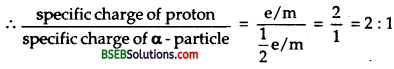Question 35. Calculate the acceleration and kinetic energy gained by an electron accelerated in a uniform electric field E and covering a distance x in the direction of force. Answer: F→ = Force on an electron in E→ = e E→ a→ = acceleration produced in the electron = 𝐹⃗ 𝑚=𝑒𝐸⃗ 𝑚 . x→ = displacement of electron along F→. If W be the work done by the force, then W = F→ . x→ = Fx = eEx.

Question 36. What led de-Broglie to the duality hypothesis for matter? Answer: According to de-Broglie a wave is associated with every moving particle and is called matter or de-Broglie wave. The following hypothesis led him to the duality hypothesiλs for matter. (i) The whole energy in the universe is in the form of matter and electromagnetic radiation. (ii) The nature loves symmetry. We know that radiation behave both as particle as well as wave, so the matter should also possess dual nature.

Question 37. Why is the wave nature not noticable in our daily observations? Answer: This is because the value of de-Broglie wavelength is very small as compared to the size of the body.

Question 38. Explain how does de-Broglie hypothesis support Bohr’s model of stationary orbits? Answer: According to de-Broglie hypothesis, λ. = hmv ……….(1) Also we know that for n electrons revolving in an orbit of radius nλ = 2πr or λ = 2πrn ……..(2) ∴ From (1) and (2), we get hm𝑣=2𝜋rn or mvr = 𝑛ℎ2π which is in accordance with Bohr’s concept of stationary orbits. Hence the statement is true.

Question 39. What is the minimum wave length of light which can cause photo-electric emission. Out of red and blue light which one can cause photo-electric emission? Answer: The minimum wavelength of light which can cause photoelectric emission is the green light. As wavelength of red light is more than that of green while that of blue light, it is lesser, so red light causes photo electric emission while blue does not.

Question 1. Derive the expression for the wave length of a particle behaving like a wave. or Prove that λ = ℎ𝑚𝑣 for matter waves. Answer: According to quantum theory of radiation, the energy (E) of a photon is given by E = hv ………(1) Also we know that if this photon is considered to be a particle of mass m, then according to theory of relativity. E = 𝑚20𝑐4+𝑝2𝑐2‾‾‾‾‾‾‾‾‾‾‾√ As m0 = rest mass a photon = 0 ∴ E = pc = mc.c = mc2 where p = mc is the momentum of photon ∴ From (1) and (2),we get mc2 = hv or mc = ℎ𝑣𝑐 or p = h(c/v) (∴ c = vλ) or p = ℎλ where λ is the wavelength of radiations or λ = ℎ𝑝 Also we know that for a moving particle of mass m and velocity υ, p = mυ. ∴ λ = ℎ𝑚υ ………..(3) Eq. (3) is the required expression which connects momentum p (which is the characteristic of particle) with the wavelength X (which is the characteristics of wave).

Question 2. Prove that the de-Broglie wavelength λ of the electrons of energy E is given by λ = 𝐡2mE√ Answer: We know that de-Broglie wavelength of electron is given by λ = ℎ𝑚υ ………(1) where m, u are the mass and velocity of electron. If E be the kinetic energy of the electron, then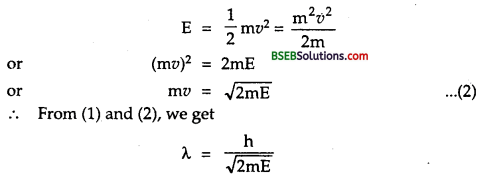Hence proved.

Question 3. What conclusion can you draw from de-Broglie relation? Answer: We know that λ = ℎ𝑚υ ……….(1) Thus it can be concluded from equation (1) that.- (i) The de-Broglie wavelength of a particle is independent of the charge or nature of the particle. (ii) The matter waves are not electromagnetic in nature. (iii) X will be smaller if the particle moves faster. (iv) Lighter the particle, greater is its de-Broglie wavelength.

Question 4. Express de-Broglie wavelength in terms of potential difference V through which it is accelerated. Answer: Let m, e be the mass and charge of an electron. V = Potential difference applied to accelerate it. If E be the energy acquired by the electron, then E = eV ………..(1) Also we know that λ = h2mE√ …….(2) from (1) and (2), we get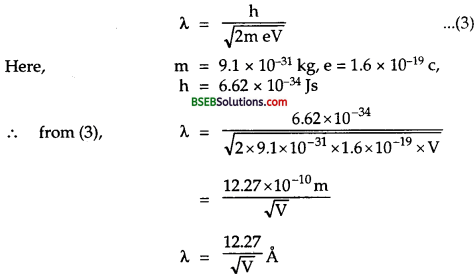which is the required relation.

Question 5. State laws of photoelectric emission. Answer: (i) No photo-electric emission takes place for a given substance if the frequency of incident radiation is lesser than the minimum frequency (called threshold frequency) whatever may be the intensity of incident radiation may be. (ii) The emission of photo electrons start as soon as light falls on metal surface i.e., process of photoelectric emission is instantaneous. (iii) The number of photo electrons emitted per second by a substance i.e., photo electric current is independent of the frequency of incident light but depends upon its intensity. (iv) The maximum kinetic energy of photoelectrons is directly proportional to the frequency of incident light but it is independent of the intensity of the light.

Question 6. Derive Enistein’s photo electric equation. Answer: According to Einstein, when a photon of energy hv falls on a metal surface, then the energy of the photon is absorbed by the free electron in the metal which is utilized in two ways : (i) A part of energy equal to work function (W) of the metal is used by the electron to over come the surface barrier and become free. (ii) The remaining part of the incident energy is carried by the photon electron in the form of maximum kinetic energy ( = 12mυ2) Thus according to the law of conservation of energy, hv = W + 12 mυmax2 ………..(1) where, υmax = maximum velocity of the photoelectron m = mass of photoelectron if v = v0, then the free electron is not emitted from the metal surface. ∴ 12 mυmax2 = 0 ……..(2) ∴ from (1) and (2), we get . hv0 = W ∴ from (1) and (3), we get hv = hv0 + 12 mυmax2 or 12 mυmax2 = hv – hv0 = h(v – v0) which is the required photo electric equation.

Question 7. In how many ways electrons from the metal surfaces can be ejected? Answer: The following are the ways of electron emission : (a) Thermionic emission (b) Photo electric emission (c) Secondary emission (d) Field emission

Question 8. Why photo electric current reduces when cathode is made mpre and more negative? Answer: The photo electric current reduces constantly when cathode is made more and more negative because when cathode is made more and move negative it repels emitted photo electrons by more and more force thereby reducing the number of electrons reaching anode.

Question 9. Prove that eV0 = h (v – v0) whre symbols have their usual meaning. What is the importance ofV0? Answer: We know that 12mυmax2 = h(v – v0) ……….(1) For just photoelectric emission, the kinetic energy of the fastest photoelectron i.e., maximum K.E. of photoelectron should be equal or more than eV0 where V0 is the stopping potential. i.e, eV0 = 12mυmax2 ……….(2) ∴ from (1) and (2), we get eV0 = h(v – v0) ……(3) from (2) we know that V0 α υmax2 i.e., the stopping potential is directly proportional to velocity or K.E. of the photo electrons. Thus V0 gives idea of the velocity and maximum energy of fastest photoelectron for first starting the photoelectric emission.

Question 10. Explain the effect of frequency of incident radiations on the photoelectric current. Answer:We know that the energy of incident radiation falling on the photoplate is given by : E = hv Thus as v is increased, the energy of incident radiation also increases. Due to higher energy of incident radiation, photoelectrons acquire more kinetic energy, thus higher is the velocity for the ejected photoelectrons. As V0 α υmax2, so higher will be the stopping potential needed to stop photo current. Titus as shown in the figure, when v1> v2 > v3, V1 > V2 > V3. But the saturation current is independent of the frequency.

Question 11. Draw a graph showing the variation of stopping potential with frequency of incident radiation in relation to photo electric effect and show that the product of the slope of the stopping potential versus frequency graph and the electronic charge gives the value of Plank’s constant. Answer: The graph between the stopping potential and frequency of incident light light is shown in the figure here :According to Einstein’s photo electric equation, 12 vmax2 = hv – hv0 ……….(1) and 12 vmax2 = eV0 ………..(2) From (1) and (2), we get eV0 = hv – hv0 …………..(3) Differentiating w.r.t. v on both sides of eq (3), we get AV0 e ΔV0Δv = h – 0 e ΔV0Δv = h ………(4) but ΔV0Δv = slope of V0 – v graph = tan θ …….(5) ∴ From (4) and (5), we get h = e x tan θ = charge x slope Hence proved.

Question 12. Mention some applications of photo electric cells. Answer: Following are the main uses of photo electric cells :

• They are used as Burglar’s alarm for houses.
• They are used for raising fire alarm.
• They are used to control temperature of chemical reactions.
• They are used as auto counters for persons entering in halls.
• They are used in automatic switching of street lights.
• They are used in photographic camera for automatic adjustment of aperture.
• They are used in a TV studio to convert light into electric current for transmission.

Question 13. The graph between the stopping potential ” V0” and frequency ‘v’ of the incident radiations of two different metal plates P and Q are shown in the figure here. (a) Which metal out of P and Q has greater value of work function? (b) What does the slope of the line depict?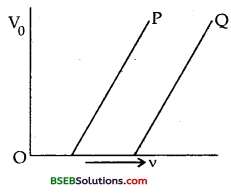Answer: (a) We know that V0 α (v – v0) Thus it is clear from the figure that the threshold frequency v0 is greater for metal Q, so the work function W0 = hv0 is greater for metal Q than P. (b) The slope of the line depict that the ratio of V0v0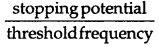is constant for both of these metals as the V – v graph is a straight line.

Question 14. Radiations of frequency 1015 Hz are incident on two photo sensitive surfaces P and Q. Following observations are made : (a) Surface P : Photoemission occurs but the photo electrons have zero kinetic energy. (b) Surface Q: Photoemission occurs and photo electrons have some kinetic energy. Which of these has a higher work function and why? If the incident frequency is slightly reduced, what will happen to photoelectron emission in two cases? Answer: (a) We know that hv = hV0 + 12 mvmax2 or hv = W + 12 mvmax2 As K.E. of P is zero, so work function of P is higher than the work function of Q because whole of the energy of the incident radiations is used to take out the free electrons from the surface P. i.e. Wp = hv for surface P i.e Wp = hv for surface P And WQ = hv – 12 mvmax2 surface Q Clearly Wp > WQ

(b) Surface P will not emit photo electrons when the frequency of incident radiation is decreased while the surface Q will emit photo electrons but their kinetic energy will decease.

Question 15. What are the characteristics of retarding potential? Answer: Following are the characteristics of retarding potential:

• It is a measure of the maximum kinetic energy of the photo electrons.
• It depends upon the nature of the metal.
• It depends upon the frequency of incident light falling on the photo sensitive material. Greater the frequency of incident light, greater is the value of stopping or retarding potential for the given surface.

Question 16. What are the properties of photons? Answer:

1. They travel with a speed of light c in vacuum.
2. They have zero rest mass.
3. They have momentum given by p = ℎλ
4. They are electrically neutral.
5. They travel in a straight line.
6. They are not deviated by electric as well as magnetic fields.
7. Their energy remains same when they travel from one medium to another.
8. They have dynamic mass given by m = ℎ𝑐λ

Question 1. Describe experiment demonstrating the wave nature of electron. Answer: Davisson and Germer experimentally verified the wave nature of electrons as predicted by de-Broglie. Experiment : Electrons from hot tungsten cathode are accelerated by a potential difference V between the cathode and anode. A narrow hole in the anode renders electrons into a fine beam and allows it to strike a nickel crystal. The electrons are scattered in all directions by the atoms in the crystal. The intensity of the electron beam scattered in a given direction is found by using a detector.

The intensity of the scattered beam can be measured for different values of ø by rotating the detector about an axis through a point O as shown below :Here ø is the angle between scattered direction of electron beam and the incident direction of beam. The graph is plotted between <|> and the intensity of scattered beam for different values of the accelerating voltages.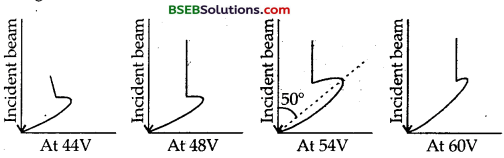From these graph we found that : (a) The intensity of scattered electrons depends upon the angle of scattering ø, (b) A kink or bump always occurs in the curve at ø = 50°. (c) The sjze of the bump goes on increasing as the accelerating voltage is increased. (d) The size of the bump becomes maximum, when the accelerating voltage is 54V. (e) The size of the bump starts decreasing with further increase in accelerating voltage. From figure 1, it follows that 20 + ø = 180° ø =180−𝜙2 For ø = 50° θ = 12 (180° – 50°) = 65° According to Bragg’s law, for first order diffraction maximum, n = 1, 2d sin θ = nλ. gives 2 d sin θ = l x λ or λ = 2d sin θ ……….(1) For nickel crystal, d = distance between atomic planes = 0.91 Å ∴ λ = 2 x 0.91 x sin 65° = 1.66 Å ……….(2) The wave nature of electron and hence de-Broglie hypotesis will be confirmed if the de-Broglie wavelength of the electrons, when accelerated by 54V comes out to be just 1.66 Å. Now de-Broglic wavelength is given by λ = h2meV√=12.27V√ Å = 12.2754√ Å = 1.65 Å ……..(3) From (2), and (3), we see that eq. (3) is in good agreement to eq. (2) hence the wave nature of electron is verified.

Numberical Problems

Question 1. Prove that if two ions of same charge and energy but different masses are passing through a uniform magnetic field, the radii of their paths are proportional to the square roots of their masses. Solution : Let m1, m2 be the masses of the two ions moving with velocities υ1 υ2 respectively. Let r1, r2 = radii of the paths of the two ions respectively. Let B uniform magnetic field. Let q be the charge on each ion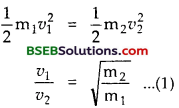in a circular path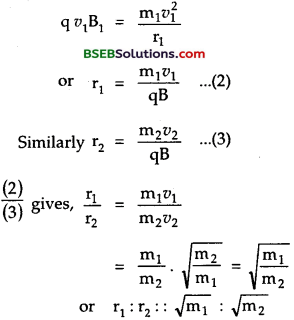Question 2. Calculate the momentum of a photon of green light (v = 6 x 1014 Hz) moving through free space. Answer: Here v = frequency of photon = 6 x 1014 Hz a = 6.62 x 10-34 Js c = 3 x 108 ms-1 P = momentum of a photon = ?

Using relation, p = ℎ𝑣𝑐, we get p = 6.62×10−34×6×10143×108 = 13.24 x 10-28 kg ms-1.

Question 3. A source of 60W emit red light of wavelength 7000 A. Calculate the number of photons emitted per second of the source. Answer: Here, P = Power of source = 60 W = 60 Js-1 λ = wavelength of light emitted = 7000 Å – 7 x 103 x 10-10m h = 6.62 x 10-34 Js Let n = number of photons emitted per second = ? E = energy of one photon = hv = ℎ𝑐λ = 6.62×10−34×1087×10−7 = 2837 x 10-19 J Using relation, n = 𝑝𝐸, we get n = 602.837×10−19 = 21.15 x 10-19

Question 4. When light of wavelength 400 nm is incident on the cathode of a photocell, the stopping potential is recorded as 6V. II the wavelength of the incident light is increased to 600 nm, calculate the new stopping potential. Answer: Here, λ 1 = wavelength of light = 400 nm = 400 x 10-9m V01= stopping potential λ1 = 6V Let W be the work function of the cathode material =? Using relation, hv = W + eV01 ……….(1) we get W = ℎ𝑐𝜆1−𝑒𝑉01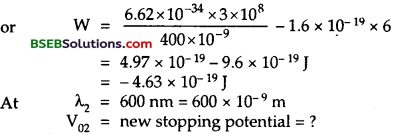∴ using eqn. (1), we get eV02 = hc𝜆2−W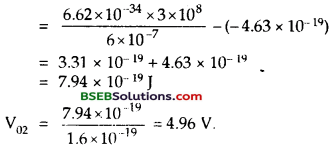Question 5. Light of wavelength 500 nm falls on a metal whose work function is 1.9 eV. Find (a) the energy of the photon (eV). (b) K.E. of the photo electrons emitted. (c) the stopping potential h = 6.62 x 10-34 Js Answer: Here, λ = wavelength of light = 500 nm = 500 x 10-9 m W = work function of the metal = 1.9 eV = 1.9 x 1.6 x 10-19 J = 3.04 x 10-19 J h – 6.62 x10-34Js

(a) Energy of photon : It is given by the relation(b) Using formula, hv = W + 12 mv2 12 mv2 = hv – W = (2.48 – 1.9) eV = .0.58 eV

(c) V0 = stopping potential = ? Using the relation, eV0 = 12 mv2, we get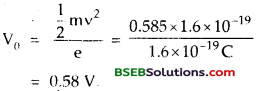Question 6. Find the wavelength of a beam of neutrons whose K.E. is 100 eV. Mass of neutron = 1.67 x 10-27kg, h = 6.62 x 10-34 Js. Answer: Here m1 = mass of neutron = 1.67 x 10-27 kg h = 6.62 x 10-34Js K.E. = 12 mv2 – 100 eV = 100 x 1.6 x 10-19J = 1.6 x 10-17 J λ = wavelength of beam of neutrons = ? we know that de-Broglie wavelength of neutrons is given by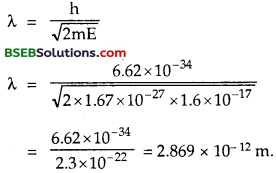Question 7. Monochromatic light of wavelength 3000 A is incident normally on a surface of area 4 cm2. If the intensity of light is 150 mW m-2, determine the rate at which photons strike the surface. Answer: Here, λ = wavelength of monochromatic light = 3000 Å = 3000 x 10-10 m A = surface area = 4 cm2 = 4 x 10-4 m2 I = intensity of light = 150 mW m-2 = 150 x 10-3 ωm-2 h = 6.62 x 10-34 Js, c = 3 x 108 ms-1 Let n be the number of photons striking/sec. = rate = ? E = energy of a photon = ℎ𝑐λ = 6.62×10−34×3×1083×10−7 = 6.62 x 10-19 J Let P = Total energy incident per sec. on the area = Power P = 1 x A = 150 x 10-3 x 4 x 10-4 = 600 x 10-7W = 6 x 10-5W n is given by n = 𝑝𝐸 = 6×10−56.62×10−19 = 9.05 x 1013 photons/s.

Question 8. A metallic surface emits electrons when irradiated by light of wavelength less than 621 nm. When it is irradiated by light of wavelength 414 nm, the stopping potential required is IV. Assuming the values of e, m, c calculate : (a) a value of h. (b) the maximum velocity of electrons emitted in second case, (c) work function of the metallic surface? Answer: Here, in 1st case, λ1 = wavelength of light t = 621 nm = 6.21 x 10-9 m In 2nd case, λ2 = 414 nm = 414 x 10-9 m; V02 = IV If E1 and E2 be the maximum kinetic energies in the two cases, then using relation E1 = 12 mυ2max = eV0 Let W be the work function the metallic surface We get E1 = eV01 = e x 0 = 0 and E2 = eV02 = 1.6 x 10-19 x 1 = 1.6 x 10-19 J ∴ using Einstein’s photoelectric equation, hv = W + E hv = W + E hc𝜆1 = W + E1 (1st case) ………(1) and hc𝜆2 = W + E2 (2nd case) …….(2) (a) (2) – (1) give, hc (1𝜆2−1𝜆1) = E2 – E1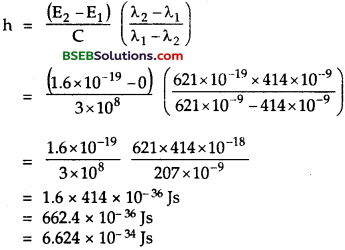(b) υmax = ? Also 12 mυ2max = eV02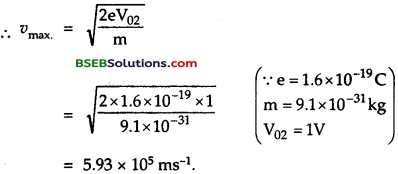(c) From equation (1), we getQuestion 9. How many photons per second does a 1W bulb emit if its efficiency is 10% and the wavelength of light emitted is 500 nm? Answer: Here, P = power of bulb = 1 W λ = Wavelength of light emitted by the bulb = 500 nm = 500 x 10-9 m = 5 x 10-7m n = no. of photons emitted/sec = ? η = efficiency of bulb = 10%Total power = P10=110 W = total energy emitted by te buld per sec. …….(1) E = hc𝜆 = energy of one photon ∴ nE = nhc𝜆 = total energy emitted by bulb/ sec. ……….(2) ∴ from (1) and (2), we get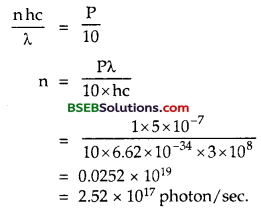Question 10. If the wavelength of the light falling on a surface is increased from 3000 Å to 3040 Å, then what will be the corresponding change in the stopping potential? Given h = 6.62 x 10-34 Js, c = 3 x 108 ms-1. Answer: Here, λ1 = 3000 Å, λ2 = 3040 Å dλ. = change in wavelength = λ1 – λ2 = 3040 – 3000 = 40 Å dλ = 40 x 10-10 m Let V0 be the stopping potential corresponding to a wavelength λ dV0 = change in stopping potential corresponding to dλ = ? e = 1.6 x 10-19C

Using Einstein’s photoelectric equation, hv = W + eV0, we get hc𝜆 = W + eV0 or eV0 = hc𝜆 – W …………(1) where W = work function of the surface differentiating (1) w.r.t. λ, we get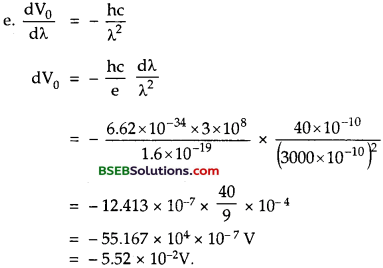Question 11. 1 mW of light of wavelength 4560 Å is incident on ; caesium surface. Calculate the photoelectric current produced, if the quantum efficiency of the surface for photoelectric emission is only 0.5%. Answer: Here, P = Power -1 mW = 10-3 W λ = wavelength = 4560 Å = 4560 x 10-10 m n = quantum efficiency = 0.5% = 51000 I = photoelectric current = ? E = energy of each photon of the incident light is given by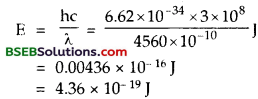Let n be the number of photons falling per second on the surface, so using relation n = 𝑝𝐸 , we get n = 1×10−34.36×10−19 = 2.294 x 10-15 As the quantum efficiency of the surface is 0.5%, hence only 0.5% of there photons produce photoelectrons. ∴ If n’ be the no. of electrons produced/sec from the surface, then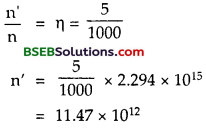using relation, I = n’e, we get I = 1147 x 1012 x 1.6 x 10-19A = 18.35 x 10-7 = 1.835 x 10-66 A = 1.84µ A

Question 12. The photo electric work functions for various metals are given below. Calculate their threshold wavelength. Which metals will not emit photoelectrons when illuminated by visible light? (a) WCu = 6.4 x 10-19J (b) WZn = 5.8 x 10-19 J (c) WK = 3.6 x 10-19J (d) WCs = 3.2 x 10-19 J Answer: Using Einstein’s photoelectric equation Emax, = hv – W ……..(1) At v = v0, Emax = 0 i.e. emitted photo electrons will have no K.E.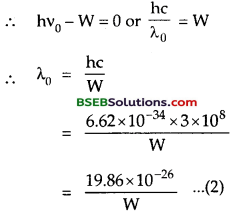We know that the range of visible light is from 4000 A to 8,000 A, hence Cu and Zn will not emit photoelectrons if illuminated by visible light as their threshold wavelength are far below the wavelength of visible light.

Question 13. What is the wavelength of a hydrogen atom moving with a velocity corresponding to mean kinetic energy for thermal equilibrium at 20°C. Answer: Here, t°C = 20°C ∴ T = absolute temperature = 273 + 20 = 293 K m = mass of hydrogen atom = 1.67 x 10-27 kg h = 6.62 x 10-34 Js. k – Boltzman’s constant = 1.38 x 10-23 JK-1. λ = de-Broglie wavelength of H-atom = ? According to kinetic theory of gases, we know that the K.E. of a gas molecule is given by 12 mv 2 = 32 kT ∴ υ = 3kTm‾‾‾‾√ ∴ p = momentum of H – atom = mυ = 3mkT‾‾‾‾‾‾√ Using, formula, λ = hm𝑣, we get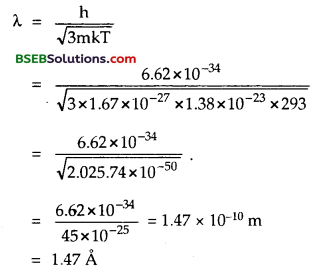Question 14. What will be de-Broglie wavelength of an electron having kinetic energy of 500 eV? Answer: Here, me = mass of electron = 9.1 x 10-31 kg. E = Kinetic energy of electron = 500 eV = 500 x 1.6 x 10-19 J. h = Plank’s constant = 6.62 x 10-34 Js. E = 12 mv2 = p22 m or p = 2mE‾‾‾‾‾√ We know that de-Broglie wavelength is given by :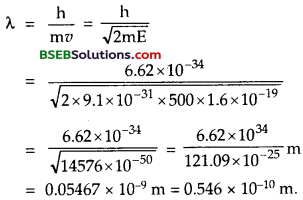Question 15. Find the potential through which an electron has to be accelerated so that the associated de-Broglie waves with moving electron has wave length I A. Answer: Here, λ de-Broglie wavelength of electron = 1 Å = l x 10-10m m = 9.1 x 10-31kg e = 1.6 x 10-19C V = ? We know that 12mv2 = eV mυ = 2meV‾‾‾‾‾‾√ Using, formula, λ = ℎ𝑚υ, we get λ = h2meV√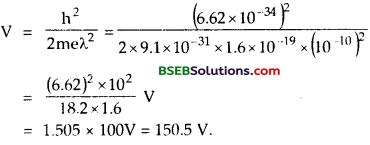Question 16. Find the uncertainty in the velocity of a proton confined in a cubical base of side 1 Å. Answer: Here, Ax = 1 Å = 10-10 m = side of box m = mass of proton = 1.67 x 10-27 kg ∆v = uncertainly in velocity of proton = ? ∆p = m∆v h = 6.62 x 10-34 Js

Using uncertainty principle,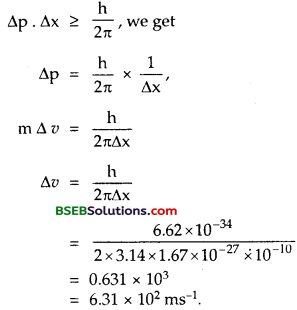Question 17. Find the de-Broglie wavelength of a proton whose kinetic energy is equal to the rest energy of an electron. Mass of proton = 1.67 x 10-27 kg, rest mass of electron, m0 = 9.1 x 10-31 kg. Answer: Here, m0 9.1 x 10-31 kg m – 1.67 x 10-27 kg λ = de Broglie wave length of proton – ? Let υp = velocity of the proton, c = 3 x 108 ms-1. According to Einstein’s mass energy relation, K.E, of proton = m0c2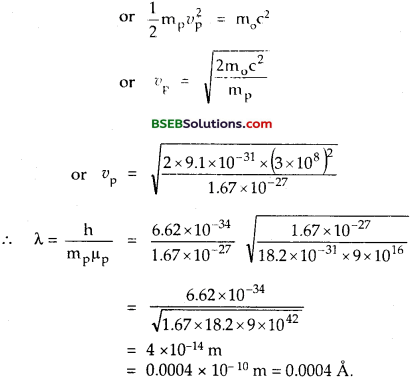Question 18. If the photo emissive surface has a threshold frequency of 4.6 x 1014 Hz, Calculate the energy of the photon in eV. Answer: Here, v0 = threshold frequency = 4.6 x 1014 Hz h = 6.62 x 10-34 Js E = energy of the photon = ? Using relation, E = hv0, we get E = 6.62 x 10-34 x 4.6 x 1014 = 30.36 x 10-20 J = 30.36×10−201.6×10−19eV = 1.897 eV.

Question 19. An electron is deflected by 1.5 mm in traversing a distance of 5 cm through an electric field of 3000 Vm-1 perpendicular to its path. If the velocity of electron is 3 x 107 ms-1, calculate e/m for the electron. deflection of electron 1.5 mm = 1.5 x 10-3 m length of plates = 5 cm = 5 x 10~2 m electric field = 3000 Vm_1 velocity of electron = 3 x 107 ms-1? Answer: Here, y = deflaction of electron = 1.5 mm = 1.5 x 10-3 m x = lenth of plates = 5 cm = 5 x 10-2 m E = elecric field = 3000 Vm-1 υ = velocity of electron = 3 x 107 ms-1 𝑒𝑚 = ? Using relationQuestion 20. Wave length of the incident light is 1.98 x 10~7 m and stopping potential is 2.5 V for photo electric emission. Find (a) threshold frequency (b) work function (c) energy of photo electrons with maximum speed. Answer: Here, λ = 1.98 x 10-7m, V0 = 2.5 V, c = 3 x 108 ms-1, h = 6.62 x 10-34 Js

(a) v0 = ? Using formula, 12 mvmax2 = hv – hv0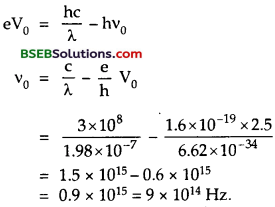(b) W = work function = ? Using relation, W = hv0, we get W = 6.62 x 10-34 x9 x 1014 J = 6.62×9×10−201.6×10−19− eV = 3.7 eV.

(c) Emax = ? Using relation,Fill In The Blanks

Question 1. Stopping potential is the measure of the ……………. of the photoelectrons and does not depend upon ………….. Answer: Maximum kinetic energy, intensity of incident light.

Question 2. In photoelectric effect experiment, photo electric current does not depend upon ………….. but depends only on the …………. of incident reduction. Answer: Frequency, intesity.

Question 3. The threshold wavelength of a photo cathode to emit photoelectrons is λ. When a radiation of wavelength X is incident on it, the average K.E. of the photoelectron is ………… Answer: h(1𝜆−1𝜆0)

Question 4. The stopping potential depends upon ………….. of incident light and …………. of metal. Answer: freqeuncy, work function.

Question 5. The maximum kinetic energy of emitted electrons in photoelectric effect is linearly dependent on the ………… of the incident radiation. Answer: freqeuncy.

Question 6. The mass of a moving photon is ………….. Answer: hc𝜆

Question 7. The photoelectric threshold frequency of a metal is uv0. When light of frequency uv0 is incident on the metal, the maximum kinetic energy of the emitted photoelectron is ………….. Answer: 3hv0

Question 8. An electron beam passes at right angles to a magnetic field of 2 x 10-3 Wb m-2. The speed of the electron is 3 x 107 ms-1. An electric field of ………….. intensity should be applied along with the magnetic field so that the path of the electron beam remains indeviated. Answer: 6 x 104 NC-1.

Question 9. An electron, photon, a neutron are accelerated through the same potential difference. The kinetic energies acquired by them will be in the ratio ………… Answer: 1 : 1 : 1.

Question 10. Light of frequency 1.5 times the threshold frequency is incident on a photo sensitive material. If the frequency of incident light is halved and the intensity is doubled, the photo current becomes ………….. Answer: Zero. [Hint : When frequency of incident light is halved then it becomes less than the threshold frequency i.e. v < v0, so no photoelectrons are emitted.]

Question 11. A photon behaves as if it had a mass equal t0 …………. and momentum equal to ……….. Answer: hvc2,lvc

Question 12. An electron is accelerated through a potential difference of 100 V. The wavelength associated with it is ………. Answer: 1.23 Å.

Question 13. An electron is accelerated through a potential difference of 104 V. The energy acquired by the electron is…….. Answer: 1.6 x 10-15J

Question 14. Out of proton, neutron, p-particle and a-particle ……….. will have the maximum de-Broglie wavelength. Answer: β – particle.

Question 15. If electrons photons are considered to be of same de- Broglie wavelength, then they will have the same ……….. Answer: momentum

Question 16. If a photon and an electron ate considered to be of same de^Broglie wavelength, then the velocity of photon is ……….. Answer: greater than that of the electron.

Question 17. An electron of mass m and charge e is accelerated from rest through a potential difference of V in vacuum. Its final velocity will be ………. Answer: 2𝑒𝑉𝑚‾‾‾‾√

Question 18. The mass of a photon at rest is Answer: zero.

Question 19. When a proton is accelerated through a potential difference of one volt, the kinetic energy gained by it is roughly equal to ………. Answer: 1 eV.

Question 20. Einstein’s photoelectric equation is expressed as ……… Answer: hv = ω0 + 12 mv2.

## Bihar Board Class 12th Physics Dual Nature of Radiation and Matter Textbooks for Exam Preparations

Bihar Board Class 12th Physics Dual Nature of Radiation and Matter Textbook Solutions can be of great help in your Bihar Board Class 12th Physics Dual Nature of Radiation and Matter exam preparation. The BSEB STD 12th Physics Dual Nature of Radiation and Matter Textbooks study material, used with the English medium textbooks, can help you complete the entire Class 12th Physics Dual Nature of Radiation and Matter Books State Board syllabus with maximum efficiency.

## FAQs Regarding Bihar Board Class 12th Physics Dual Nature of Radiation and Matter Textbook Solutions

#### Can we get a Bihar Board Book PDF for all Classes?

Yes you can get Bihar Board Text Book PDF for all classes using the links provided in the above article.

## Important Terms

Bihar Board Class 12th Physics Dual Nature of Radiation and Matter, BSEB Class 12th Physics Dual Nature of Radiation and Matter Textbooks, Bihar Board Class 12th Physics Dual Nature of Radiation and Matter, Bihar Board Class 12th Physics Dual Nature of Radiation and Matter Textbook solutions, BSEB Class 12th Physics Dual Nature of Radiation and Matter Textbooks Solutions, Bihar Board STD 12th Physics Dual Nature of Radiation and Matter, BSEB STD 12th Physics Dual Nature of Radiation and Matter Textbooks, Bihar Board STD 12th Physics Dual Nature of Radiation and Matter, Bihar Board STD 12th Physics Dual Nature of Radiation and Matter Textbook solutions, BSEB STD 12th Physics Dual Nature of Radiation and Matter Textbooks Solutions,
Share: